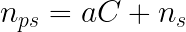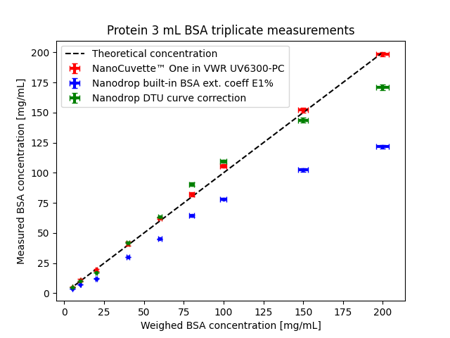# What is Hand's Law?

## Hand's Law states that the refractive index of a protein solution is directly proportional to its concentration.

Traditionally, protein concentration measurement utilizes the Beer-Lambert law, which states that the concentration is directly proportional to the absorbance shift at a fixed wavelength. The practical implementation of this can be tricky.

An alternative way to calculate protein concentrations is to exploit Hand's Law. The refractive index of a protein solution is directly proportional to its concentration.Eq. 1 Hand's Law

nₚₛ is the refractive index of the protein solution, nₛ is the refractive index of the solvent, is a proportionality constant, sometimes called the refractive index increment and is the concentration of the solution. 

The refractive index increment, a, is an important parameter for concentration determination of a protein. One can dissolve a protein in a known solvent and perform a series of dilutions to calculate this value a from the refractive index measurements of the dilutions.

An example of an experimental procedure demonstrating protein quantification using a spectrophotometer and exploiting Hand's Law can be found here. One of the results from this paper is shown below in Figure 1.Fig. 1 Protein concentration determination. The red points (NanoCuvette™ One in VWR UV6300-PC) are calculated using a procedure that utilizes Hand's Law. Conversely, the blue points (Nanodrop built-in BSA ext. coeff E1%) utilize the Beer-Lambert law.

With a UV-Vis spectrophotometer, using the NanoCuvette™ One in conjunction with SpectroWorks™ software, concentration measurements can be made from sample sizes as small as 0.5μL and a wide concentration range of 0.02 - 300 mg/mL.

References

### Contact

Copenhagen Nanosystems ApS,
Hørmarken 2, DK-3520 Farum, Denmark
Tel: +45 36 99 27 46

www.cphnano.com